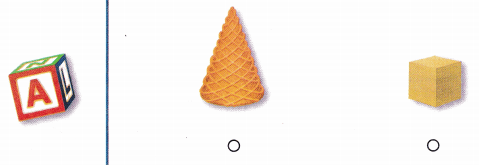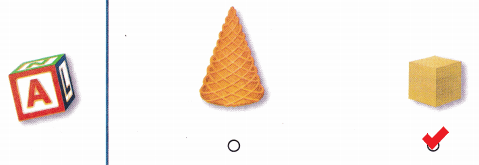# Texas Go Math Kindergarten Module 18 Assessment Answer Key

Refer to our Texas Go Math Kindergarten Answer Key Pdf to score good marks in the exams. Test yourself by practicing the problems from Texas Go Math Kindergarten Module 18 Assessment Answer Key.

## Texas Go Math Kindergarten Module 18 Assessment Answer Key

Concepts and Skills

Question 1.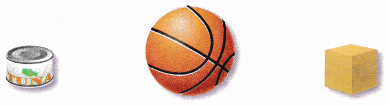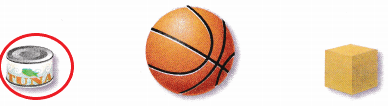Explanation:
I drew a circle around the object that is shaped like a cylinder.

Question 2.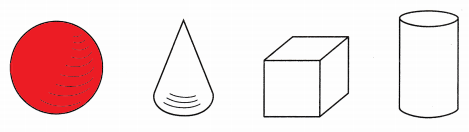Explanation:
I colored the sphere among all the shapes.

Question 3.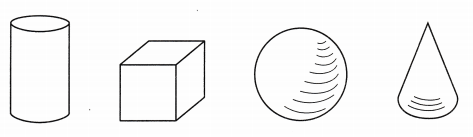Explanation:
I colored a cube among all the shapes.

Question 4.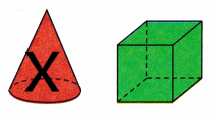Explanation:
A cone has curved surface
I marked X on the shape that has a cured surface.

Question 5.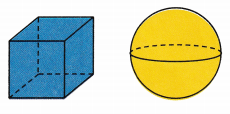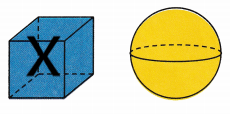Explanation:
A cube has flat surfaces
So, i marked X on the shape that has flat surfaces.

Directions
1. Circle the object that is shaped like a cylinder. TEKS K.6.B 2. Color the sphere. TEKS K.6.E 3. Color the cube. TEKS K.6.E 4. Mark on X on the shape that has a curved surface. T.E.K.S K.6.E 5. Mark on X on the shape that has
flat surfaces. TEKS K.6.E.

Question 6.Explanation:
I colored the cube and drew a circle around the two-dimensional shape that is the same shape as the flat surface.

Question 7.Explanation:
I colored the cylinder and drew a circle around the two-dimensional shape that is the same shape as the flat surface.

Texas Test Prep

Question 8.